# Review Z Test Ci 1

17. Jul 2009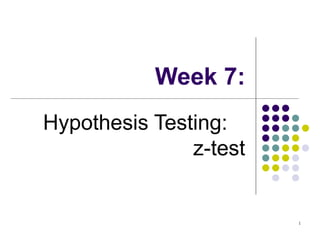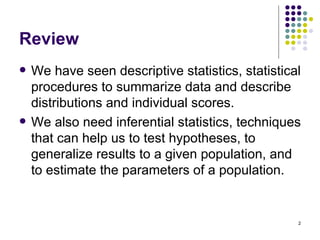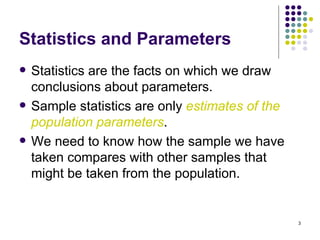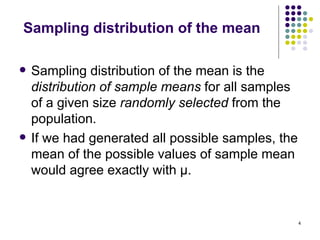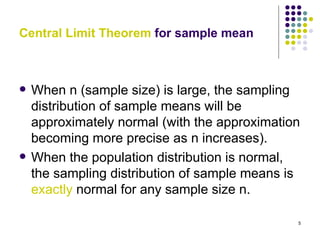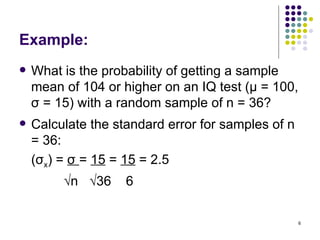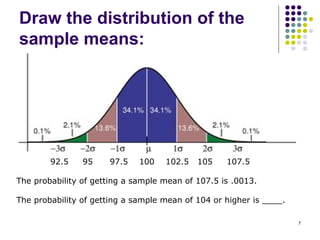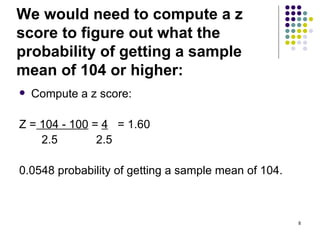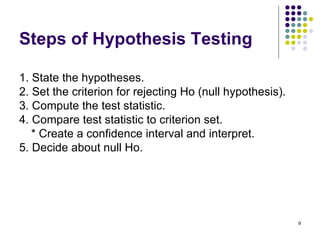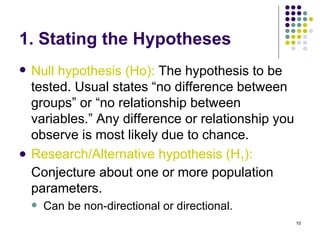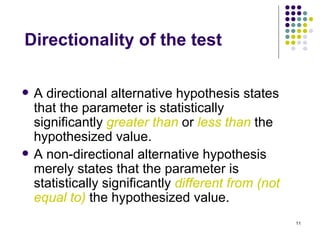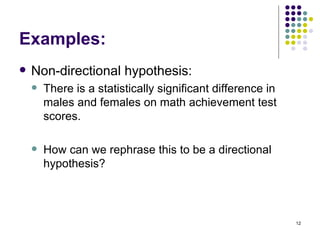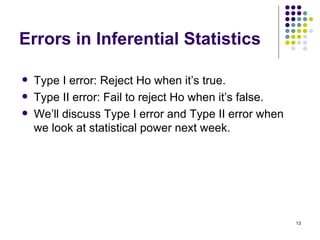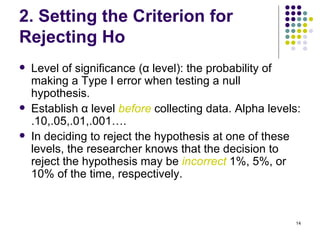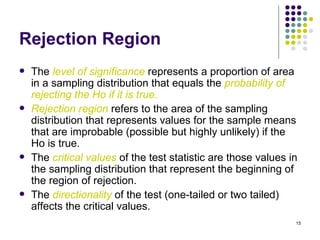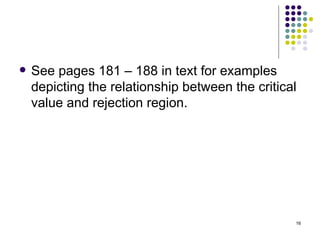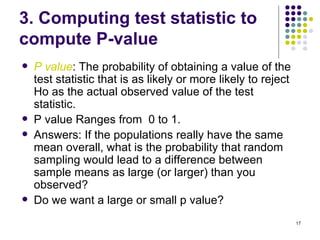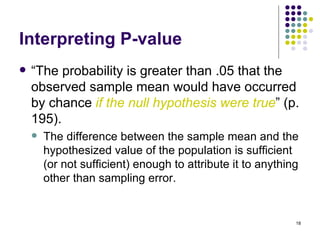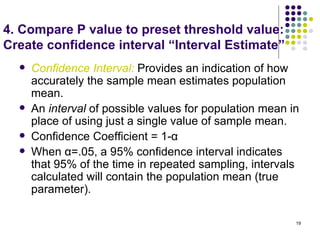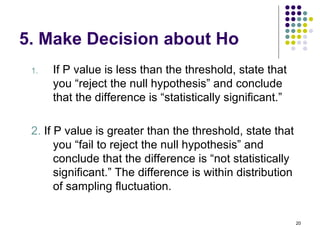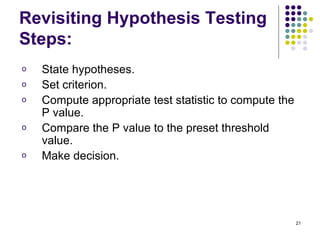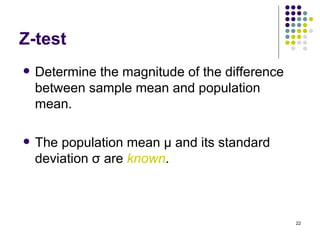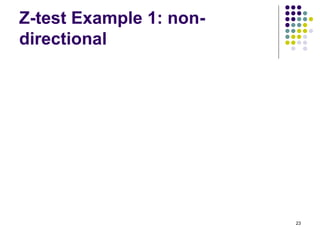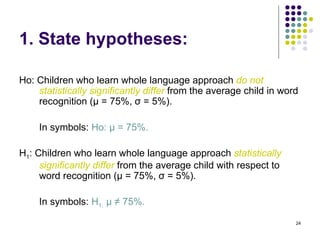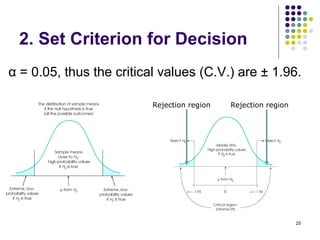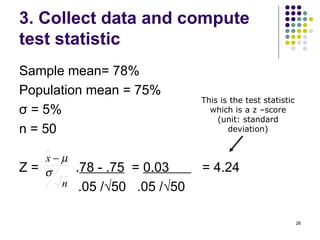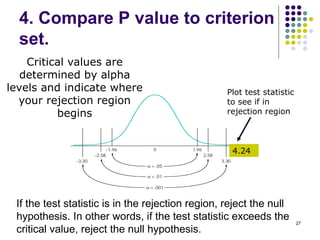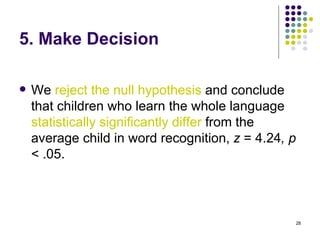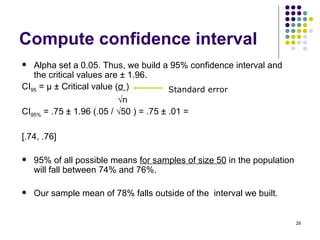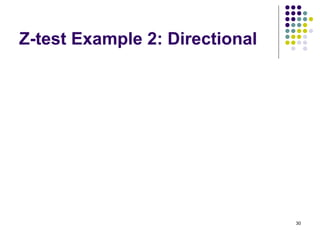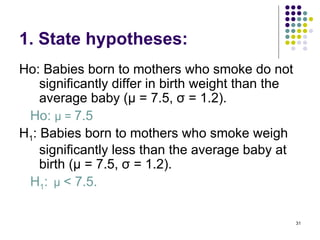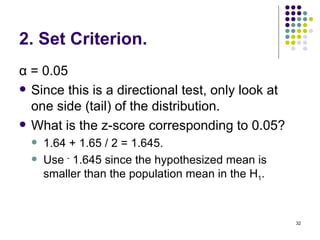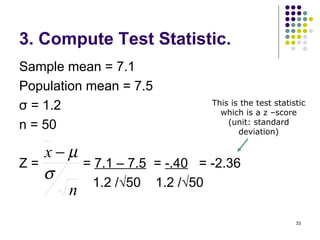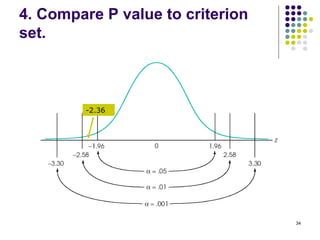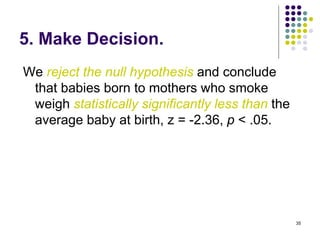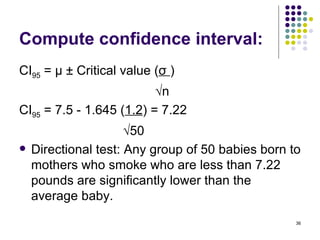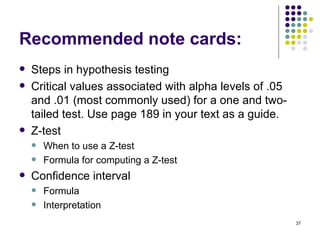1 von 37

### Review Z Test Ci 1

• 1. Week 7: Hypothesis Testing: z-test 1
• 2. Review  We have seen descriptive statistics, statistical procedures to summarize data and describe distributions and individual scores.  We also need inferential statistics, techniques that can help us to test hypotheses, to generalize results to a given population, and to estimate the parameters of a population. 2
• 3. Statistics and Parameters  Statistics are the facts on which we draw conclusions about parameters.  Sample statistics are only estimates of the population parameters.  We need to know how the sample we have taken compares with other samples that might be taken from the population. 3
• 4. Sampling distribution of the mean  Sampling distribution of the mean is the distribution of sample means for all samples of a given size randomly selected from the population.  If we had generated all possible samples, the mean of the possible values of sample mean would agree exactly with μ. 4
• 5. Central Limit Theorem for sample mean  When n (sample size) is large, the sampling distribution of sample means will be approximately normal (with the approximation becoming more precise as n increases).  When the population distribution is normal, the sampling distribution of sample means is exactly normal for any sample size n. 5
• 6. Example:  What is the probability of getting a sample mean of 104 or higher on an IQ test (μ = 100, σ = 15) with a random sample of n = 36?  Calculate the standard error for samples of n = 36: (σx) = σ = 15 = 15 = 2.5 √n √36 6 6
• 7. Draw the distribution of the sample means: 92.5 95 97.5 100 102.5 105 107.5 The probability of getting a sample mean of 107.5 is .0013. The probability of getting a sample mean of 104 or higher is ____. 7
• 8. We would need to compute a z score to figure out what the probability of getting a sample mean of 104 or higher:  Compute a z score: Z = 104 - 100 = 4 = 1.60 2.5 2.5 0.0548 probability of getting a sample mean of 104. 8
• 9. Steps of Hypothesis Testing 1. State the hypotheses. 2. Set the criterion for rejecting Ho (null hypothesis). 3. Compute the test statistic. 4. Compare test statistic to criterion set. * Create a confidence interval and interpret. 5. Decide about null Ho. 9
• 10. 1. Stating the Hypotheses  Null hypothesis (Ho): The hypothesis to be tested. Usual states “no difference between groups” or “no relationship between variables.” Any difference or relationship you observe is most likely due to chance.  Research/Alternative hypothesis (H1): Conjecture about one or more population parameters.  Can be non-directional or directional. 10
• 11. Directionality of the test  A directional alternative hypothesis states that the parameter is statistically significantly greater than or less than the hypothesized value.  A non-directional alternative hypothesis merely states that the parameter is statistically significantly different from (not equal to) the hypothesized value. 11
• 12. Examples:  Non-directional hypothesis:  There is a statistically significant difference in males and females on math achievement test scores.  How can we rephrase this to be a directional hypothesis? 12
• 13. Errors in Inferential Statistics  Type I error: Reject Ho when it’s true.  Type II error: Fail to reject Ho when it’s false.  We’ll discuss Type I error and Type II error when we look at statistical power next week. 13
• 14. 2. Setting the Criterion for Rejecting Ho  Level of significance (α level): the probability of making a Type I error when testing a null hypothesis.  Establish α level before collecting data. Alpha levels: .10,.05,.01,.001….  In deciding to reject the hypothesis at one of these levels, the researcher knows that the decision to reject the hypothesis may be incorrect 1%, 5%, or 10% of the time, respectively. 14
• 15. Rejection Region  The level of significance represents a proportion of area in a sampling distribution that equals the probability of rejecting the Ho if it is true.  Rejection region refers to the area of the sampling distribution that represents values for the sample means that are improbable (possible but highly unlikely) if the Ho is true.  The critical values of the test statistic are those values in the sampling distribution that represent the beginning of the region of rejection.  The directionality of the test (one-tailed or two tailed) affects the critical values. 15
• 16. See pages 181 – 188 in text for examples depicting the relationship between the critical value and rejection region. 16
• 17. 3. Computing test statistic to compute P-value  P value: The probability of obtaining a value of the test statistic that is as likely or more likely to reject Ho as the actual observed value of the test statistic.  P value Ranges from 0 to 1.  Answers: If the populations really have the same mean overall, what is the probability that random sampling would lead to a difference between sample means as large (or larger) than you observed?  Do we want a large or small p value? 17
• 18. Interpreting P-value  “The probability is greater than .05 that the observed sample mean would have occurred by chance if the null hypothesis were true” (p. 195).  The difference between the sample mean and the hypothesized value of the population is sufficient (or not sufficient) enough to attribute it to anything other than sampling error. 18
• 19. 4. Compare P value to preset threshold value: Create confidence interval “Interval Estimate”  Confidence Interval: Provides an indication of how accurately the sample mean estimates population mean.  An interval of possible values for population mean in place of using just a single value of sample mean.  Confidence Coefficient = 1-α  When α=.05, a 95% confidence interval indicates that 95% of the time in repeated sampling, intervals calculated will contain the population mean (true parameter). 19
• 20. 5. Make Decision about Ho 1. If P value is less than the threshold, state that you “reject the null hypothesis” and conclude that the difference is “statistically significant.” 2. If P value is greater than the threshold, state that you “fail to reject the null hypothesis” and conclude that the difference is “not statistically significant.” The difference is within distribution of sampling fluctuation. 20
• 21. Revisiting Hypothesis Testing Steps: o State hypotheses. o Set criterion. o Compute appropriate test statistic to compute the P value. o Compare the P value to the preset threshold value. o Make decision. 21
• 22. Z-test  Determine the magnitude of the difference between sample mean and population mean.  The population mean μ and its standard deviation σ are known. 22
• 23. Z-test Example 1: non- directional 23
• 24. 1. State hypotheses: Ho: Children who learn whole language approach do not statistically significantly differ from the average child in word recognition (µ = 75%, σ = 5%). In symbols: Ho: µ = 75%. H1: Children who learn whole language approach statistically significantly differ from the average child with respect to word recognition (µ = 75%, σ = 5%). In symbols: H1: µ ≠ 75%. 24
• 25. 2. Set Criterion for Decision α = 0.05, thus the critical values (C.V.) are ± 1.96. Rejection region Rejection region 25
• 26. 3. Collect data and compute test statistic Sample mean= 78% Population mean = 75% This is the test statistic σ = 5% which is a z –score (unit: standard n = 50 deviation) x−µ Z= σ .78 - .75 = 0.03 = 4.24 n .05 /√50 .05 /√50 26
• 27. 4. Compare P value to criterion set. Critical values are determined by alpha levels and indicate where Plot test statistic your rejection region to see if in begins rejection region 4.24 If the test statistic is in the rejection region, reject the null hypothesis. In other words, if the test statistic exceeds the 27 critical value, reject the null hypothesis.
• 28. 5. Make Decision  We reject the null hypothesis and conclude that children who learn the whole language statistically significantly differ from the average child in word recognition, z = 4.24, p < .05. 28
• 29. Compute confidence interval  Alpha set a 0.05. Thus, we build a 95% confidence interval and the critical values are ± 1.96. CI95 = μ ± Critical value (σ ) Standard error √n CI95% = .75 ± 1.96 (.05 / √50 ) = .75 ± .01 = [.74, .76]  95% of all possible means for samples of size 50 in the population will fall between 74% and 76%.  Our sample mean of 78% falls outside of the interval we built. 29
• 30. Z-test Example 2: Directional 30
• 31. 1. State hypotheses: Ho: Babies born to mothers who smoke do not significantly differ in birth weight than the average baby (µ = 7.5, σ = 1.2). Ho: µ = 7.5 H1: Babies born to mothers who smoke weigh significantly less than the average baby at birth (µ = 7.5, σ = 1.2). H1: µ < 7.5. 31
• 32. 2. Set Criterion. α = 0.05  Since this is a directional test, only look at one side (tail) of the distribution.  What is the z-score corresponding to 0.05?  1.64 + 1.65 / 2 = 1.645.  Use - 1.645 since the hypothesized mean is smaller than the population mean in the H1. 32
• 33. 3. Compute Test Statistic. Sample mean = 7.1 Population mean = 7.5 σ = 1.2 This is the test statistic which is a z –score (unit: standard n = 50 deviation) x−µ Z= = 7.1 – 7.5 = -.40 = -2.36 σ 1.2 /√50 1.2 /√50 n 33
• 34. 4. Compare P value to criterion set. -2.36 34
• 35. 5. Make Decision. We reject the null hypothesis and conclude that babies born to mothers who smoke weigh statistically significantly less than the average baby at birth, z = -2.36, p < .05. 35
• 36. Compute confidence interval: CI95 = μ ± Critical value (σ ) √n CI95 = 7.5 - 1.645 (1.2) = 7.22 √50  Directional test: Any group of 50 babies born to mothers who smoke who are less than 7.22 pounds are significantly lower than the average baby. 36
• 37. Recommended note cards:  Steps in hypothesis testing  Critical values associated with alpha levels of .05 and .01 (most commonly used) for a one and two- tailed test. Use page 189 in your text as a guide.  Z-test  When to use a Z-test  Formula for computing a Z-test  Confidence interval  Formula  Interpretation 37